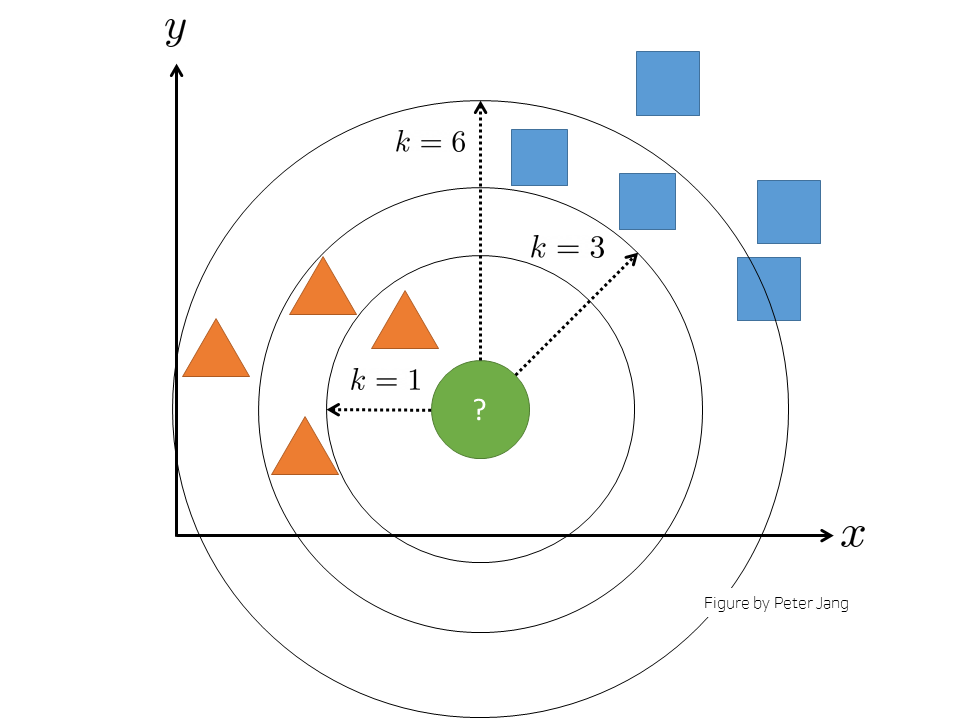# Introduction to k-NN Classification with Python

Note: This post is a “motivation” post for newcomers to data science. It covers just enough information about k-NN classification for beginners to get started on their journey.

# Introduction

The world of data science is becoming complex — especially with a wide variety of machine learning algorithms, methods, and fields of application expanding at a very fast pace.

As a data scientist, I realized that I sometimes over-complicate solutions for no reason, such as implementing a convolutional neural network for a certain task when implementing a more ‘relaxed’ machine learning algorithm would work and even perform better in certain cases.

Artificial neural networks, which I believe take many hours of studying and practice — are very interesting to work with and can give you fascinating results if you implement them correctly. However, I believe that it is much more effective and efficient taking simple routes at certain times.

I would like to take this chance to share a “convenient” machine learning algorithm — the k-NN classification algorithm.

# Brief History of the k-NN Algorithm

In 1951, statisticians Evelyn Fix and Joseph Hodges, Jr. broke new ground with their paper: “Discriminatory Analysis. Nonparametric Discrimination: Consistency Properties” [PDF].

Then, many researchers expanded on this study, which resulted in an interesting machine learning algorithm: the k-nearest neighbors algorithm (k-NN).

# What is the k-NN Algorithm? Why k?

k-NN is a supervised machine learning algorithm that heavily depends on the proximity of data. It essentially “believes” that similar data exist in close proximity — like “clusters”.

It is like how countries with similar traditions and cultures are in close proximity in groups called “continents”.

Here, the k represents the number of nearest neighbors’ “group names”, which is used to assign a “group” to the current data.k-NN Classification with k nearest neighbors

For the remainder of the post, let:

• k be a positive integer that represents the number of “nearest neighbors”
• f be the “function” that represents the k-NN algorithm
• x be the input

The k-NN algorithm can be used for two different purposes:

• Classification
• Regression

## k-NN Classifier

With the k-NN algorithm, we can develop a classifier such that if we input our data x into the algorithm f (i.e. f(x)), then the output of the function will be the predicted “class membership” or “cluster” of the input data.

The algorithm basically calculates the distances between the input data and the dataset that the classifier was trained with and outputs the predicted “class”.

## k-NN Regressor

If we input our data x into the algorithm f , then the output of the function will be the average of the values of k nearest neighbors

We will be focusing on k-NN Classification in this post

# Demonstration

As a demonstration, we will be developing a binary classification model that is able to classify between a smartphone and a tablet.

This is basically asking the k-NN model:

“Hey, is the device with height ‘h’ and width ‘w’ a smartphone or a tablet?”

and the k-NN model we are about to develop will predict whether the device is a smartphone or a tablet.

## Setting up

We will be using the most popular programming language in the machine learning field: Python

For instructions setting up Python for data science, please check out:

• Setting up Python for Data Science in 2021 (Currently Under Revision…)

Installing and Importing Packages
For this demonstration, we will need matplotlib, scikit-learn, and pandas.

Import data

Visualize the raw data using matplotlib

As we can see, the blue plots represent phone data and the orange plots represent tablet data.

Just like how we can “classify” the phone data and tablet data with our common sense by looking at the graph, we can make the k-NN classifier do the same!

Before we call our k-NN classifier and train it, let’s combine both phone and tablet data into two lists: height and width

## Process Data for Training

We can label them with numbers:

• 0 for tablet
• 1 for phone

k-NN Classifier

• Let’s now develop our k-NN classifier using a well-known data science library called scikit-learn
• In the code below, we basically call our friend k-NN classifer over from scikit-learn and name it kn
• Then, we “fit” our k-NN classifier
• Finally, we test the accuracy of our classifier using the score function — also from scikit-learn!

Hold up, what is fitting?

Check out:

• What does it mean to fit a model anyway? [Diamond Age]
• Why do we need to fit a k-nearest neighbors classifier? [Stack Exchange]

Testing our friend k-NN Classifier

• Yay! We developed our k-NN Classifier using scikit-learn
• Although we got a score of the model, let’s test it using real inputs!

The graph is similar to our raw data scatter plot — except there are two extra plots.

As a human being looking at the scatter plot, it is instinctively obvious that the green plot is a phone and a red plot is a tablet, but would our k-NN classifier also have the same instinct? — Let’s find out.

Testing our k-NN Classifier with Inputs

I coded a very simple Python script that just prints out if our k-NN classifier classifies the input as a phone or a tablet:

# Applications

The k-NN classification algorithm can be applied to a wide variety of problems:

• Weather forecasting — is it going to rain today or not?
• Genetics — does this DNA (x) resemble COVID-19 or not?
• … and so many more!

Try out different datasets with your own processed data.
Advanced features of the k-NN classifier can be absolutely studied by oneself.

# Things to think about

• Picking a value for k
• Pros and Cons of k-NN
• The mathematical viewpoint of k-NN (Hint: Euclidean distance)